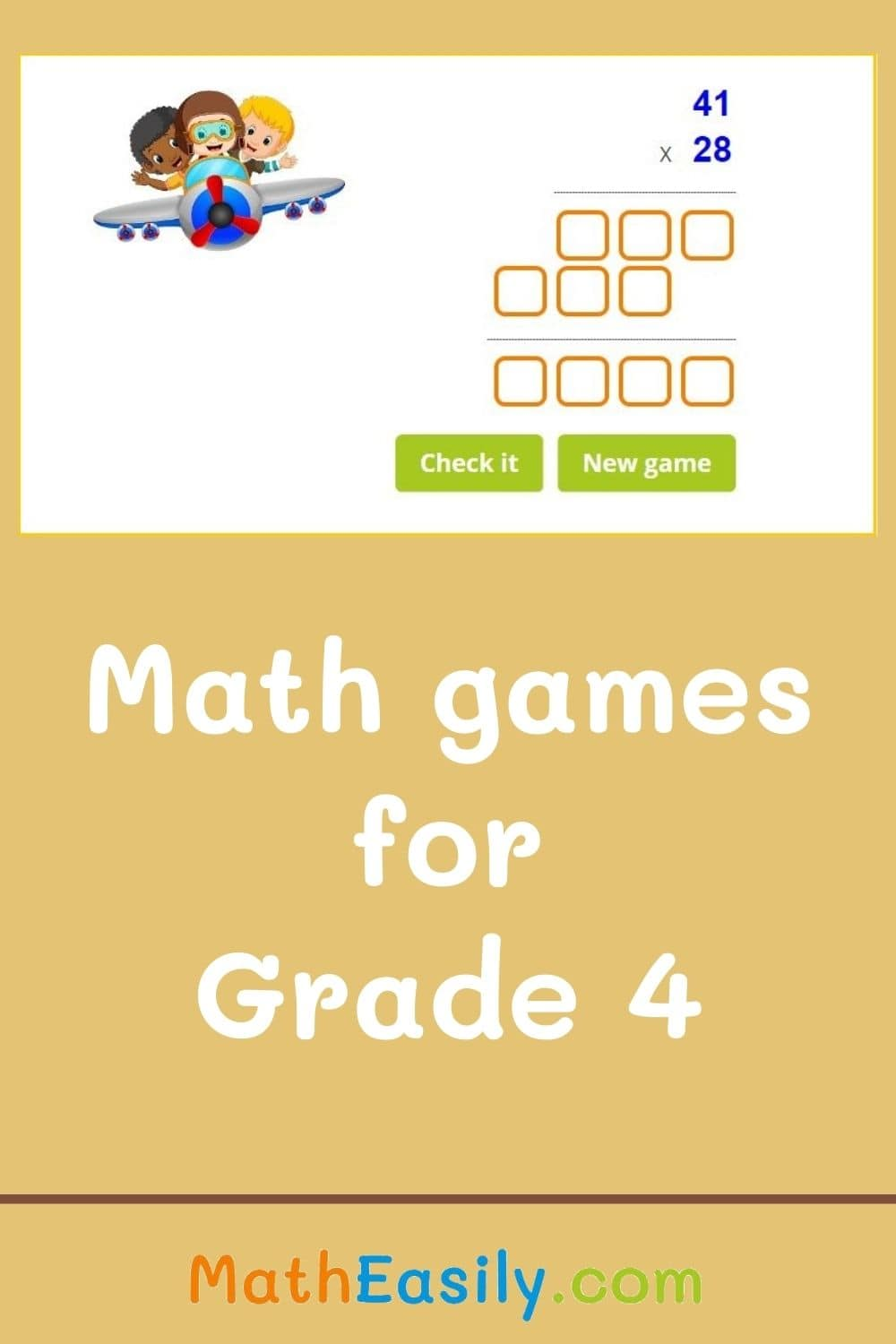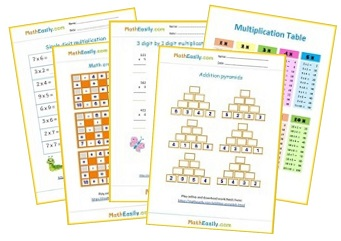﻿ 100+ Free Math Games for Grade 4 ONLINE Practice

Page No. 1048

Free Math Games online > 4th Grade Math

# Free Math Games for Grade 4

Play Online Math Games for Grade 4. Check results, find mistakes. Addition, subtraction, multiplication, division and shapes. You may freely use any of our free 4th grade math games and exercises in your classroom or for home practice.

All these interactive math games for 4th grade are accompanied by worksheets on which kids can practice a certain skill. You can find all our 4th Grade math worksheets here.

## Math games for grade 4 ONLINE free

### 3 digit addition with regrouping

You can choose between 2 options: 3 digit addition games with or without regrouping. Play free online math games for grade 4.
Play now

### 3 digit subtraction games

Practice 3 digit subtraction online. Free online math games for 4th graders: 3 digit subtraction with or without regrouping games.
Play now

### Math picture puzzles

Play interactive picture puzzles. Learn addition, subtraction and multiplication. Solve the picture equations.
Play now

Play addition magic squares online, download magic squares worksheets. Magic squares puzzles improve memory and logical thinking.
Play now

### Identifying fractions

Identify basic fractions of shapes. Students have to recognize which fraction corresponds to a given picture. Learn fractions easily with these 4th grade math games.
Play now

### Fractions on a number line

Select the fraction that corresponds to the given point on the number line. Placing fractions on a number line´ games.
Play now

Play now

### 2 digit subtraction online games

Practice column subtraction up to 100 with our colored worksheets, play online math games for 4th graders.
Play now

## Free math games for 4th graders

Play free math learning games for 4th graders, that teach multiplication and division:

### Matchstick puzzles

Move one match to fix the equation. A new matchstick puzzle every day. Download match puzzles with answers in PDF.

Play now

### Multiplication flash cards

Online multiplication flash cards (up to 12) help kids to learn multiplication. More than 160 tasks available. Free worksheets.

Play now

#### Math Christmas puzzles

Play interactive math games for Christmas. Download printable math Christmas puzzles in PDF to enjoy the festive time.

Play now

#### Math Halloween puzzles

Solve Halloween math puzzles. Download math Halloween emoji puzzles in PDF. Play Hallowen math games online.

Play now

### Division flash cards

Math games for grade 4: division flash cards 1-12. Division card game can be played anywhere and anytime.

Play now

### Multiplication pyramids

Play multiplication pyramid games, learn to multiply within 100, 300 or 500. Download free worksheets.

Play now

### Factor game: BINGO

Click the correct number which can substitute the question mark. Get 5 stars in row or column.

Play now

### 2 digit by 1 digit multiplication

Learn how to multiply two by one digit numbers. Play free online math games for 4th grade.

Play now

Play now

### 2 digit multiplication

Learn 2 digit by 2 digit multiplication online. Popular online math games for 4th grade. Worksheets included.

Play now

### 3 digit by 2 digit multiplication

Learn to multiply 3 digit by 2 digit numbers.

Play now

### Three digit multiplication

Learn how to multiply 3x3 digit numbers. Watch the tutorial, find mistakes. Download worksheets.

Play now

## 4th grade math games printable

Download printable math games for 4th graders below. The worksheet has two pages, the first one is the worksheet and the other one contains also the answers. Each worksheet contains a link to the related game, where kids can do many math exercises for 4th grade. Get instant access to all our math worksheets for Grade 4 here.### Math problems for 4th graders

These free math games for Grade 4 teach kids addition, subtraction, multiplication and division.

Our young mathematicians can work on improving their math problem solving skills as they find their way through each maths puzzle.

So, when you need a quick, easy (and quiet!) activity for your kids, here are online math games for grade 4.

## Free 4th grade math games for the classroom

Learning Math is about practicing. Play these free 4th grade games to practice, what you should already know from previous school years:

Play interactive math games for grade 4. Move the digits on the left side into the correct positions.
Play now

Need more exercises? Check these online 3rd Grade Math games.Free Math Worksheets